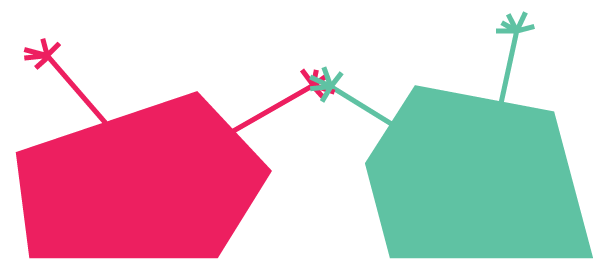# Area Of Similar Polygons

Geometry Level 2The sum of the areas of two similar polygons is 65 square units. If their perimeters are 12 units and 18 units, respectively, what is the area of the larger polygon?

×